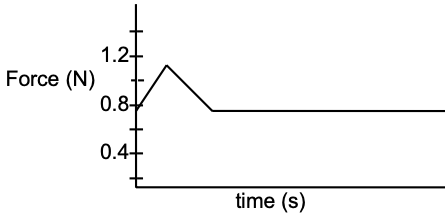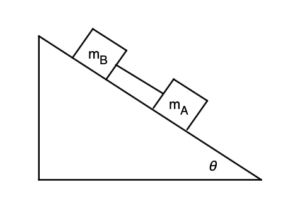## Physics UBQ

Supercharge Your Learning with 1000+ AP Level Physics Problems

###### MCQ

A ball of mass m is fastened to a string. The ball swings at constant speed in a vertical circle of radius R with the other end of the string held fixed. Neglecting air resistance, what is the difference between the string’s tension at the bottom of the circle and at the top of the circle?

###### MCQ

Two satellites are in circular orbits around Earth. Satellite A has speed vA . Satellite B has an orbital radius nine times that of satellite A. What is the speed of satellite B?

###### GQ

Find the net gravitational force on a 2.0 kg sphere midway between a 4.0 kg sphere and a 7.0 kg sphere that are 1.2 m apart.

###### GQ

A 2.2 kg ball on the end of a 0.35 m long string is moving in a vertical circle. At the bottom of the circle, its speed is 5.3 m/s. Find the tension in the string.

###### GQ

A person’s back is against the inner wall of spinning cylinder with no support under their feet. If the radius is R, find an expression for the minimum angular speed so the person does not slide down the wall. The coefficient of static friction is µs.

Note: If you haven’t studied angular velocity \omega yet, just find the linear velocity v.

###### GQ

The distance from earth to sun is 1.0 AU. The distance from Saturn to sun is 9 AU. Find the period of Saturn’s orbit in years. You can assume that the orbits are circular.

###### GQ

Find the escape speed from a planet of mass 6.89 x 1025 kg and radius 6.2 x 106 m.

###### MCQ

A block starts at rest on a frictionless inclined track which then turns into a circular loop of radius R and is vertical. In terms of R and constants, find the minimum height h above the bottom of the loop the block must start from so it makes it around the loop.

###### FRQ

A satellite circling Earth completes each orbit in 132 minutes.

1. Find the altitude of the satellite.
2. What is the value of g at the location of this satellite?
###### MCQ

A car is safely negotiating an unbanked circular turn at a speed of 17 m/s on dry road. However, a long wet patch in the road appears and decreases the maximum static frictional force to one-fifth of its dry-road value. If the car is to continue safely around the curve, by what factor would the it need to change the original velocity?

###### GQ

An airplane can safely bank when subjected to a centripetal acceleration of 8 g’s. If the airplane flies at a constant speed of 400 m/s, how long does it take to make a 180° turn?

###### GQ

An Olympic bobsled team goes through a horizontal curve at a speed of 120 km/hr. If the radius of curvature is 10.0 m, what is the apparent weight the crew experiences-express in terms of mg.

###### GQ

What is a man’s apparent weight at the equator if his weight is 500 N? The earth’s radius is 6.37 x 106 m.

###### MCQ

A rock is whirled on the end of a string in a horizontal circle of radius R with a constant period T. If the radius of the circle is reduced to R/3, while the period remains T, what happens to the centripetal acceleration (ac) of the rock?

###### MCQ

Two identical object rests on a platform rotating at constant speed. Object A is at distance of half the platform’s radius from the center. Object B lays at edge of the platform. Assuming the platform continues rotating at the same speed, how does the centripetal force of the two objects compare?

###### GQ

What force would have to be applied to start a 12.3 kg wood block moving on a surface with a static coefficient of friction of 0.438?

###### GQ

A 1509 g wood block is being pulled by the force meter at a constant velocity. Using the graph below find:

1. The force applied to get the wood block moving
2. The static coefficient of friction
3. The kinetic coefficient of friction###### FRQTwo blocks made of different materials, connected by a thin cord, slide down a plane ramp inclined at an angle \theta to the horizontal. If the coefficients of friction are µA = .2 and µB = .3 and if mA = mB = 5.0 kg determine

1. The acceleration of the blocks.
2. The tension in the cord, for an when the angle \theta = 32°.

## Submit A Question